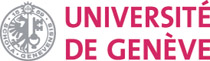Martin J. Gander Section de Mathématiques 2-4 rue du Lièvre, CP 64 CH-1211 Genève Martin.Gander(at)unige.ch   Fax: +41 22 379 11 76Home Research Teaching Consulting Personal

 Seminars Genève: - Analyse numérique McGill: - CSE - Applied Mathematics Conference Ascona 2013

 Available projects Postdoctoral: - upon request Doctoral degree: - upon request Master thesis: - upon request

 :: Fast non-conforming interface projections An example of a fast advancing front technique to compute L2 projections from an arbitrary triangular grid onto another arbitrary one for non-conforming (e.g. mortar) methods. To obtain the Matlab code, see below. Check also out the 3d case, and the case for contact problems, where the interfaces two dimensional, but in 3d, and not at precisely the same geometrical location.Below is the short Matlab code which does the projection: it is explained in the paper "An algorithm with optimal complexity for non-matching grid projections", M.J. Gander and C. Japhet, Proceedings of the 18th international conference of Domain Decomposition methods, 2008. The complete demo code in Matlab with an example can be downloaded here, and also the slides of the corresponding presentation with several helpful illustrations. ``` ``` function M=InterfaceMatrix(Na,Ta,Nb,Tb); % INTERFACEMATRIX projection matrix for nonmatching triangular grids % M=InterfaceMatrix(Na,Ta,Nb,Tb); takes two triangular meshes Ta % and Tb with associated nodal coordinates in Na and Nb and % computes the interface projection matrix M bl=; % bl: list of triangles of Tb to treat bil=; % bil: list of triangles Ta to start with bd=zeros(size(Tb,1)+1,1); % bd: flag for triangles in Tb treated bd(end)=1; % guard, to treat boundaries bd(1)=1; % mark first triangle in b list. M=sparse(size(Nb,2),size(Na,2)); while length(bl)>0 bc=bl(1); bl=bl(2:end); % bc: current triangle of Tb al=bil(1); bil=bil(2:end); % triangle of Ta to start with ad=zeros(size(Ta,1)+1,1); % same as for bd ad(end)=1; ad(al)=1; n=[0 0 0]; % triangles intersecting with neighbors while length(al)>0 ac=al(1); al=al(2:end); % take next candidate [P,nc,Mc]=Intersect(Nb(:,Tb(bc,1:3)),Na(:,Ta(ac,1:3))); if ~isempty(P) % intersection found M(Tb(bc,1:3),Ta(ac,1:3))=M(Tb(bc,1:3),Ta(ac,1:3))+Mc; t=Ta(ac,3+find(ad(Ta(ac,4:6))==0)); al=[al t]; % add neighbors ad(t)=1; n(find(nc>0))=ac; % ac is starting candidate for neighbor end end tmp=find(bd(Tb(bc,4:6))==0); % find non-treated neighbors idx=find(n(tmp)>0); % take those which intersect t=Tb(bc,3+tmp(idx)); bl=[bl t]; % and add them bil=[bil n(tmp(idx))]; % with starting candidates Ta bd(t)=1; end ``` ```

Home Research Teaching Consulting Personal